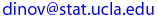Ivo Dinov
UCLA Statistics, Neurology, LONI
, Math/PIC
 Courses SOCR Ivo Dinov's Home SiteMap Software Contact

STAT 35
Winter 2005

Interactive and Computational Probability

Department of Statistics

## Instructor: Ivo Dinov

Homework 2
Due Date: Wednesday, Feb 09, 2005

Please, submit your homework before lecture on the due date. Correct solutions to any 4 out of the 4 problems carry full credit. See the HW submission rules. On the front page include the following header

• (HW_2_1)  A computer consulting firm presently has bids out on three projects. Let Ai = { awarded project i }, for i = 1, 2, 3, and suppose that P(A1) = 0.22, P(A2) = 0.25, P(A3) = 0.28, P(A1 ∩ A2) = 0.11, P(A1 ∩ A3) = 0.05, P(A2 ∩ A3) = 0.07, P(A1 ∩ A2 ∩ A3) = 0.01. Express in words each of the following events and compute the probability of each event.
(a) A1 ∪ A
(b) A'1 ∩ A'2
(c) A1 ∪ A2 ∪ A3
(d) A'1 ∩ A'2 ∩ A'3
(e) A'1 ∩ A'2 ∩ A3
(f) (A'1 ∩ A'2) ∪ A3

• (HW_2_2)  An insurance company offers four different deductible levels – none, low, medium, and high – for its homeowner’s policyholders and three different levels – low, medium, and high – for its automobile policyholders. The accompanying table gives proportions for the various categories of policyholders who have both types of insurance. For example, the proportion of individuals with both low homeowner’s deductible and low auto deductible is 0.06 (6% of all such individuals). Suppose an individual having both types of policies is randomply selected.
 Homeowner’s

 Auto N L M H L 0.04 0.06 0.05 0.03 M 0.07 0.10 0.20 0.10 H 0.02 0.03 0.15 0.15

(a) What is the probability that the individual has a medium auto deductible and a high homeowner’s deductible?
(b) What is the probability that the individual has a low auto deductible? A low homeowner’s deductible?
(c) What is the probability that the individual is in the same category for both auto and homeowner’s deductibles?
(d) Based on your answer in part (c), what is the probability that the two categories are different?
(e) What is the probability that the individual has at least one low de-ductible level?
(f) Using the answer in part (e), what is the probability that neither deductible level is low?

• (HW_2_3)  A card is drawn from a statndard well-shuffled deck of 52 playing cards.  What is the theoretical probability of the event A = {the drawn card is a king or a club}? In this applet an outcome of a King is recorded in the first variable Y and corresponds to Y=13; the second variable Z corresponds to the suit of the card with a Club, ♣, represented by Z=0.  Using this Card-Experiment from the SOCR resource perform 20 experiments and determine the proportion of the outcomes the event A was observed. This would correspond to the emperical probability (the chance) of the event A. How close are the observed and the theoretical proabilities for the event A? Would the discrepancy between these increase or dicrease if the number of hands drawn increases? Experiment and report!

• (HW_2_4)  Suppose we need to validate that a coin given to us is fair. We toss the coin 6 times independently and observe only one Head. If the coin was fair P(Head)=P(Tail)=0.5 and we would expect about 3 Heads and 3 Tails. Under these fair coin assumptions what is the (theoretical) probability that only 1 Head is observed in 6 tosses? Use the Binomial Coin Experiment to:
• Emprerically compute the odds (chances) of observing one Head in 6 fair-coin-tosses (run 100 experiments and record the number of them that contain exactly 1 Head);
• Emperically estimate the Bias of the coin we have tested. Experiment with tossing 30 coins at a time. You should change the p-value=P(Head), run experiments and pick a value on the X-axis that the emperical distribution (red-histogram) peaks at. Perhaps you want this peak X value to be close to the observed 1-out-of-6 Head-count for the original test of the coin. Include some graph snapshots and some brief discussion regaring your experiments and findings.

 Ivo Dinov's Home http://www.stat.ucla.edu/~dinov Visitor number, since Jan. 01, 2002 © I.D.Dinov 1997-2005
Last modified on by